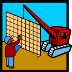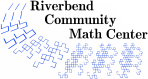# Grid Grab Game

### Description

In the Grid Grab Game, students work with fractions, decimals and percents simultaneously. Students will recognize that an amount on a 100 grid can be represented in all three formats and will learn to add rational numbers accurately. Assessment is embedded with use of the 100 grid model and shading.

### Preparation Time

approximately five minutes for students to retrieve materials needed and label spinner sections

### Activity Time

approximately 10 to 15 minutes per game played

### Topics

• Addition of fractions, decimals, and percents
• Conversion of fractions, decimals, and percents
• Comparison of fractions, decimals and percents

### Goals

• Participants will develop and deepen conceptual understanding of rational numbers.
• Participants will increase their familiarity with using a 100 grid as a model for rational numbers.

### Materials

• Game rules for each pair (or group of 4 if playing in teams)
• A recording sheet for each group
• Colored pencils (at least five colors)
• A page of hundred grids for each student
• Spinner handouts and 2 paper clips for each group

### Prerequisites

• Students should have been introduced to representations of positive rational numbers using hundred grids, including rational numbers greater than 1.

### Credits

NCTM Illuminations Activity Fraction Models: A computer activity that has students practice with models for fractions, decimals, and percents http://illuminations.nctm.org/ActivityDetail.aspx?ID=11

### Authors

Cheryl A. Nix, M.S.

### National Common Core Standards

 4.Focus.2 Develop an understanding of fraction equivalence, addition and subtraction of fractions with like denominators, and multiplication of fractions by whole numbers. 4.NF.5 Express a fraction with denominator 10 as an equivalent fraction with denominator 100, and use this technique to add fractions with those denominators. 4.NF.6 Use decimal notations for fractions with denominators 10 or 100. 4.NF.7 Compare two decimals to hundredths by reasoning about their sizes. 5.Focus.2 Fluently add, subtract, multiply, and divide multi-digit numbers and make sense of standard algorithms with decimals using models for fractions and decimals. 5.NBT.1 Recognize that in a multi-digit whole number, a digit in one place represents ten times what it represents in the place to its right. (e.g. 700/70 = 10). 5.NF.3 Interpret a fraction as division of the numerator by the denominator. Solve word problems involving division of whole numbers leading to answers in the form of fractions or mixed numbers, e.g. by using visual fraction models or equations to represent the problem. 6.Focus.2 Exhibit conceptual understanding and algorithmic fluency with rational numbers and the four operations. 6.RP.3 Use ratio and rate reasoning to solve real-world and mathematical problems (make tables, solve unit rate problems, work with percents, and convert measurement units).
Riverbend Community Math Center
hello@riverbendmath.org
http://riverbendmath.org
(574) 339-9111This work placed into the public domain by the Riverbend Community Math Center.